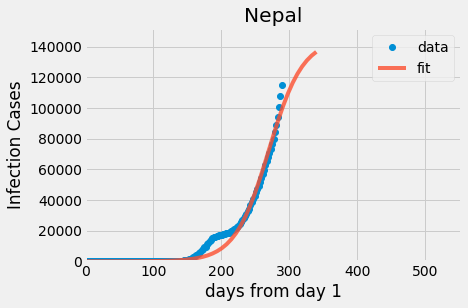# Nepal - Covid-19 Prediction models using different ML algorithms

Posted on May 26, 2021, 12:44 p.m., 564, by: sagarI am using a sigmoidal function to fit the historical data of Covid-19 and predict or forecast. And also use LinearRegression and RandomForestRegressor to predict. I thought of a sigmoidal function first because China's data resembled a sigmoidal shape. Therefore, I try to fit sigmoid functions onto Nepal also.

import pandas as pd
import numpy as np
import matplotlib.pyplot as plt
plt.style.use('fivethirtyeight')

df1.columns
Step-2: Subsetting only those rows that have "NPL" in the "location" column and plot y-axis total_cases over x-axis as day-count.

Nepal_df = df1[df1['location']=='Nepal'].groupby('date')[['total_cases','total_deaths']].sum()
Nepal_df['day_count'] = list(range(1,len(Nepal_df)+1))
ydata = Nepal_df.total_cases
xdata = Nepal_df.day_count
Nepal_df['rate'] = (Nepal_df.total_cases-Nepal_df.total_cases.shift(1))/Nepal_df.total_cases
Nepal_df['increase'] = (Nepal_df.total_cases-Nepal_df.total_cases.shift(1))
# Nepal_df = Nepal_df[Nepal_df.total_cases>100]

plt.plot(xdata, ydata, 'o')
plt.title("Nepal")
plt.ylabel("Population Infected")
plt.xlabel("Days")
plt.show()

Step-3: Sigmoidal Function:
from scipy.optimize import curve_fit
import pylab

def sigmoid(x,c,a,b):
y = c*1 / (1 + np.exp(-a*(x-b)))
return y

xdata = np.array([1, 2, 3,4, 5, 6, 7])
ydata = np.array([0, 0, 13, 35, 75, 89, 91])

popt, pcov = curve_fit(sigmoid, xdata, ydata, method='dogbox',bounds=([0.,0., 0.],[100,2, 10.]))
print(popt)

x = np.linspace(-1, 10, 50)
y = sigmoid(x, *popt)

pylab.plot(xdata, ydata, 'o', label='data')
pylab.plot(x,y, label='fit')
pylab.ylim(-0.05, 105)
pylab.legend(loc='best')
pylab.show()

Sigmoid function, Here is a snap of how I learnt to fit Sigmoid Function - y = c/(1+np.exp(-a*(x-b))) and 3 coefficients [c, a, b]:

• c - the maximum value (eventual maximum infected people, the sigmoid scales to this value eventually)
• a - the sigmoidal shape (how the infection progress. The smaller, the softer the sigmoidal shape is)
• b - the point where the sigmoid start to flatten from steepening (the midpoint of sigmoid, when the rate of increase start to slow down)

Step-4: Since our dataset doesn't have a recovered cases column so I am gonna import and read the next dataset

# Extract list of values of 'recovered' column and insert that column to our first data-subset with proper arrangement.
# Since few pairs of row doesn't exist in column of new dataset, so we gonna manually add values referencing other sites.
list(df2.iloc[172, 4:])
input_values = [0, 0, 0, 0, 0, 0, 0, 0, 0, 0, 0, 0, 0, 0, 0, 0, 0, 0, 0, 0, 0, 0, 0, 0, 0, 0, 0, 0, 0, 0, 0, 0, 0, 0, 0, 0, 0, 0, 0, 0, 0, 0, 0, 0, 1, 1, 1, 1, 1, 1, 1, 1, 1, 1, 1, 1, 1, 1, 1, 1, 1, 1, 1, 1, 1, 1, 1, 1, 1, 1, 1, 1, 1, 1, 1, 1, 1, 1, 1, 1, 1, 1, 1, 1, 1, 1, 1, 1, 1, 1, 1, 1, 1, 1, 1, 1, 1, 1, 1, 1, 1, 1, 1, 1, 1, 1, 1, 1, 2, 2, 2, 4, 4, 4, 7, 10, 11, 12, 16, 16, 16, 16, 16, 16, 16, 16, 16, 16, 22, 22, 31, 31, 31, 33, 33, 35, 35, 36, 36, 36, 36, 37, 45, 49, 70, 70, 87, 112, 155, 183, 187, 206, 219, 220, 221, 266, 278, 290, 333, 365, 467, 488, 584, 674, 861, 877, 913, 974, 1041, 1158, 1167, 1186, 1402, 1578, 1772, 2148, 2224, 2338, 2650, 2698, 2834, 3013, 3134, 3194, 4656, 5320, 6143, 6415, 6547, 6811, 7499, 7752, 7891, 8011, 8442, 8589, 10294, 10328, 11025, 11249, 11534, 11637, 11695, 11868, 12477, 12684, 12840, 12947, 13053, 13128, 13754, 13875, 14021, 14248, 14399, 14492, 14603, 14961, 15026, 15156, 15389, 15814, 16313, 16353, 16493, 16664, 16728, 16837, 17077, 17201, 17335, 17495, 17580, 17700, 17964, 18214, 18350, 18631, 18806, 19119, 19504, 20073, 20242, 20555, 20822, 21410, 22178, 23290, 24207, 25561, 27127, 28941, 30677, 32964, 33882, 35700, 36672, 37524, 38697, 39576, 40638, 41706, 42949, 43820, 45267, 46233, 47238, 48061, 49954, 50411, 51866, 53013, 53898, 54640, 55371, 56428, 57389, 60696, 62740, 64069, 65202, 67542, 68668, 71343, 73023, 74252, 75804, 77277, 78780, 80954]
len(input_values)

step-5: Creating new subset with required columns and rows only:

in_df1 = df1[df1['location']=='Nepal'].groupby('date')[['total_cases', 'new_cases', 'total_deaths', 'new_deaths', 'total_tests']].sum().reset_index(False)
in_df1['recovered']= np.array(input_values).copy()
in_df1['Active']=in_df1['total_cases']-in_df1['new_deaths']-in_df1['recovered']
# in_df1 = in_df1[in_df1.Active>=20]

in_df1.isnull().sum()

Step-6: Sigmoidal fitting

in_df1['day_count'] = list(range(1, len(in_df1)+1))
in_df1['increase'] = (in_df1.total_cases-in_df1.total_cases.shift(1))
in_df1['rate'] = (in_df1.total_cases-in_df1.total_cases.shift(1))/in_df1.total_cases

def sigmoid(x,c,a,b):
y = c*1 / (1 + np.exp(-a*(x-b)))
return y

xdata = np.array(list(in_df1.day_count)[::2])
ydata = np.array(list(in_df1.total_cases)[::2])

# population=29136808
# popt, pcov = curve_fit(sigmoid, xdata, ydata, method='dogbox',bounds=([0., 0., 0.], [population, 6, 180.]))
# print(popt)

# Step-7: Prediction Using Manual sigmoidal fitting:

import pylab

est_a = 145000
est_b = 0.04
est_c = 270
x = np.linspace(-1, Nepal_df.day_count.max()+50, 50)
y = sigmoid(x,est_a,est_b,est_c)
pylab.plot(xdata, ydata, 'o', label='data')
pylab.plot(x,y, label='fit',alpha = 0.8)
pylab.ylim(-0.05, est_a*1.05)
pylab.xlim(-0.05, est_c*2.05)
pylab.legend(loc='best')
plt.xlabel('days from day 1')
plt.ylabel('Infection Cases')
plt.title('Nepal')
pylab.show()

print('model start date:',Nepal_df[Nepal_df.day_count==1].index)
print('model start infection:',int(Nepal_df[Nepal_df.day_count==1].total_cases))
print('model fitted max infection at:',int(est_a))
print('model sigmoidal coefficient is:',round(est_b,2))
print('model curve stop steepening, start flattening by day:',int(est_c))
print('which is date:', Nepal_df[Nepal_df.day_count==int(est_c)].index)
print('model curve flattens by day:',int(est_c)*2)

display(Nepal_df.tail(3))